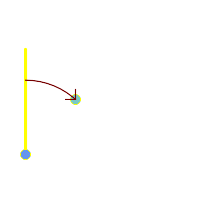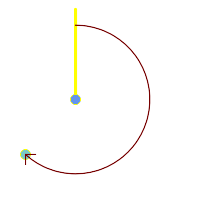## Name

ST_Azimuth — Returns the north-based azimuth of a line between two points.

## Synopsis

`float ST_Azimuth(`geometry origin, geometry target`)`;

`float ST_Azimuth(`geography origin, geography target`)`;

## Description

Returns the azimuth in radians of the target point from the origin point, or NULL if the two points are coincident. The azimuth angle is a positive clockwise angle referenced from the positive Y axis (geometry) or the North meridian (geography): North = 0; Northeast = π/4; East = π/2; Southeast = 3π/4; South = π; Southwest 5π/4; West = 3π/2; Northwest = 7π/4.

For the geography type, the azimuth solution is known as the inverse geodesic problem.

The azimuth is a mathematical concept defined as the angle between a reference vector and a point, with angular units in radians. The result value in radians can be converted to degrees using the PostgreSQL function `degrees()`.

Azimuth can be used in conjunction with ST_Translate to shift an object along its perpendicular axis. See the `upgis_lineshift()` function in the PostGIS wiki for an implementation of this.

Availability: 1.1.0

Enhanced: 2.0.0 support for geography was introduced.

Enhanced: 2.2.0 measurement on spheroid performed with GeographicLib for improved accuracy and robustness. Requires PROJ >= 4.9.0 to take advantage of the new feature.

## Examples

Geometry Azimuth in degrees

```SELECT degrees(ST_Azimuth( ST_Point(25, 45),  ST_Point(75, 100))) AS degA_B,
degrees(ST_Azimuth( ST_Point(75, 100), ST_Point(25, 45) )) AS degB_A;

dega_b       |     degb_a
------------------+------------------
42.2736890060937 | 222.273689006094
```Blue: origin Point(25,45); Green: target Point(75, 100); Yellow: Y axis or North; Red: azimuth angle.Blue: origin Point(75, 100); Green: target Point(25, 45); Yellow: Y axis or North; Red: azimuth angle.